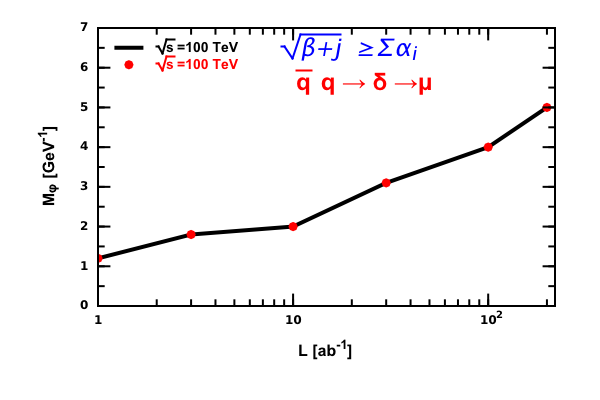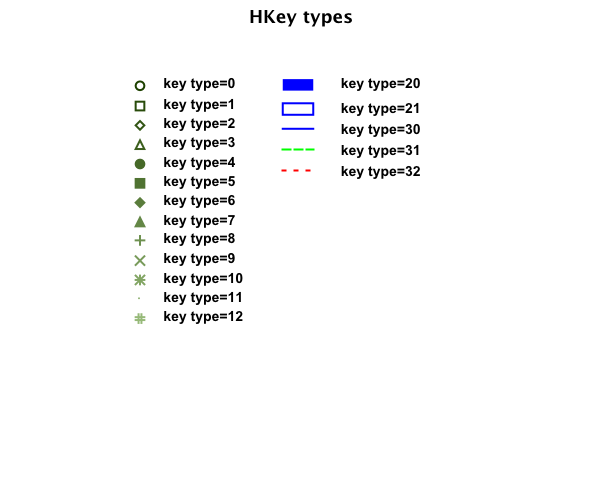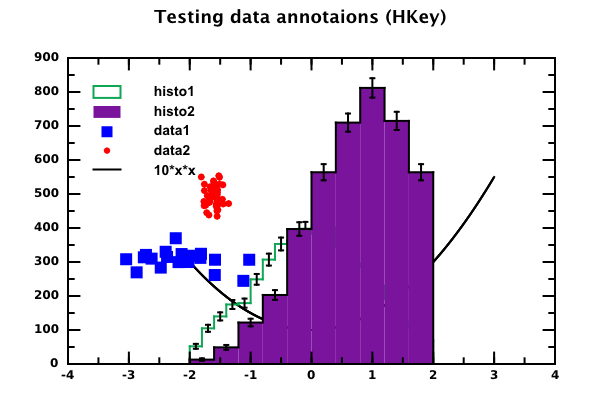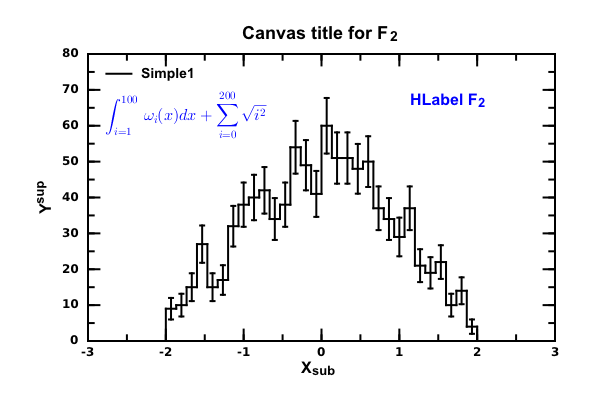# DMelt:Plots/8 Plot Annotations

Limitted access. First login to DataMelt if you are a full DataMelt member. Then login to HandWiki as a user.

# Plot annotations

Data can be annotated using interactive legends and labels. In this section we will briefly discuss main components to annotate data points. For annotations, use strings. You can include Greek or math symbols as explained below.

# Greek symbols

All labels and legends or any string which can be shown on a canvas can contain Greek letters. The table below shows how to encode Greek letters:

<html>

Entity nameAppearanceEntity nameAppearanceEntity nameAppearance
&alpha;α&beta; β &gamma; γ
&delta; δ &epsi; &epsi; &zeta; ζ
&eta; η &theta; θ &thetav; &thetav;
&iota; ι &kappa; κ &lambda; λ
&mu; μ &nu; ν &xi; ξ
&pi; π &piv; ϖ &rho; ρ
&sigma; σ &sigmav; &sigmav; &tau; τ
&upsi; &upsi; &phi; φ &phiv; &phiv;
&chi; χ &psi; ψ &omega; ω
&Gamma; Γ &Delta; Δ &Theta; Θ
&Lambda; Λ &Xi; Ξ &Pi; Π
&Sigma; Σ &Upsi; &Upsi; &Phi; Φ
&Psi; Ψ &Omega; Ω

</html>

# Mathematical operators

All labels and legends or any string which can be shown on a canvas can contain math operators:

<html>

Entity nameAppearanceEntity nameAppearanceEntity nameAppearance
&perp;&plusmn; ± &or;
&and;&le;&ge;
&equiv;&ap; &ap; &ne;
&sub;&sube;&sup;
&supe;&isin;&larr;
&rarr;&uarr;&darr;
&harr;&lArr;&rArr;
&uArr;&dArr;&iff; &iff;
&forall;&exist;&inf; &inf;
&nabla;

</html>

You can find more symbols in Unicode Consortium for HTML symbols.

Alternatively, you can use characters using numbers. See the unicode table unicode table When defining a Unicode character, use "&", followed by "#", then a number and then close it with a semi-column. For example, the string:

s="W^{ &#8723;}"

will show a W boson with minus and plus sign, <html>∓</html>.

# Simple labels

The package jhplot.shapes can be used to display several geometrical primitives, pictures, including the text labels. All of this works together with thejhplot.HPlot canvas. You cannot interact with the mouse using such labels.

# Interactive labels

You can annotate your figure on thejhplot.HPlot canvas putting interactive labels, so you can move them around with the mouse and edit.

The text labels can be set manually or via the label property window (click on the interactive label to bring up a dialog). Subscripts and superscripts in the text should be included as for the standard LaTeX syntax.

• "X^{2}" will be shown as $\displaystyle{ X^{2} }$
• "X_{2}" will be displayed as $\displaystyle{ X_{2} }$

To indicate over-line, use the reserved word #bar{}. For example, to display $\displaystyle{ \overline{X+Y} }$, use #bar{X+Y}. To display a square root $\sqrt{X+Y}$, use #sqrt{X+Y}.

To make a space between characters, use the usual space. To make a back-space, use the predefined keyword #bs2{}. This creates a backspace with the width of one character. To make a smaller backspace, equal to 1/2 of the character width, use #bs1{}.

One can mix this syntax to show complicated mathematical equations in the labels.

Symbols must be encoded using the entity reference notation as shown in the above sections. For example, the Greek "omega" should be written as "ω"

This is a small example that shows data points and create labels using mathematical symbols and Greek letters.

The Jython / Python script that creates this figure is given below. You can use the Java syntax to create a similar plot.

which shows this image:# Interactive legends

Interactive legends are used to annotate data points, functions or histograms. Be default, once data are plotted, data annotation is also displayed using title of the data (getTitle()) as a text for the key. Still, one can set legends for data manually. Look at the API of thejhplot.HKey class.

In this example, we have disabled automatic legends and made legends using thejhplot.HKey class. We plot 3 different objects, which we pass to the HKey to make legends.

The position of legends are set automatically. This example generates the figure below:The legend can show symbols, lines or filled areas, and in fact, their positions can be set manually. In fact, to make a legend, there is no need to use input plotted objects.

Here we created an interactive label (type=0) at the position x=15,y=100 which shows a symbol (open circle). The type of the symbol (0) is set using the method "setKey".

Legends are fully customizable. In the example below we change many attributes of legendsThe figure was generated with the example code shown below. Here we change many default attributes of the standard legends using the Python codding:

# LaTeX equations

If you are usingjhplot.HPlot, you can show complex LaTeX equations using the classjhplot.HLabelEq. This example shows how to make such a label using NDC coordinate system. It also shows to to put an interactive label

The output is shown here: